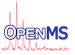OpenMS
RansacModel< ModelT > Class Template Reference

Generic plug-in template base class using 'Curiously recurring template pattern' (CRTP) to allow for arbitrary RANSAC models (e.g. linear or quadratic fits). More...

#include <OpenMS/MATH/MISC/RANSACModel.h>

Inheritance diagram for RansacModel< ModelT >:
[legend]

## Public Types

typedef std::pair< double, double > DPair

typedef std::vector< DPairDVec

typedef DVec::const_iterator DVecIt

typedef std::vector< double > ModelParameters

## Public Member Functions

ModelParameters rm_fit (const DVecIt &begin, const DVecIt &end) const
fit a model and return its parameters More...

double rm_rsq (const DVecIt &begin, const DVecIt &end) const
Returns the R-squared of the data applied to the model (computed on-the-fly). More...

double rm_rss (const DVecIt &begin, const DVecIt &end, const ModelParameters &coefficients) const
calculates the residual sum of squares of the input points according to the model More...

DVec rm_inliers (const DVecIt &begin, const DVecIt &end, const ModelParameters &coefficients, double max_threshold) const

## Detailed Description

### template<class ModelT = int> class OpenMS::Math::RansacModel< ModelT >

Generic plug-in template base class using 'Curiously recurring template pattern' (CRTP) to allow for arbitrary RANSAC models (e.g. linear or quadratic fits).

An actual model should derive from this class using CRTP (see RansacModelLinear for an example) and implement the *_impl() functions.

## ◆ DPair

 typedef std::pair DPair

## ◆ DVec

 typedef std::vector DVec

## ◆ DVecIt

 typedef DVec::const_iterator DVecIt

## ◆ ModelParameters

 typedef std::vector ModelParameters

## ◆ rm_fit()

 ModelParameters rm_fit ( const DVecIt & begin, const DVecIt & end ) const
inline

fit a model and return its parameters

## ◆ rm_inliers()

 DVec rm_inliers ( const DVecIt & begin, const DVecIt & end, const ModelParameters & coefficients, double max_threshold ) const
inline

calculates the squared residual of each input point vs. the model. All points with an error <= max_threshold, are accepted as inliers and returned.

## ◆ rm_rsq()

 double rm_rsq ( const DVecIt & begin, const DVecIt & end ) const
inline

Returns the R-squared of the data applied to the model (computed on-the-fly).

Takes as input a standard vector of a standard pair of points in a 2D space.

Parameters
 begin Iterator to first pair end Past-end iterator to last pair
Returns
: R-squared value